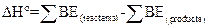Chapter 9, Problem 9.108QPGeneral Chemistry - Standalone boo...

11th Edition
Steven D. Gammon + 7 others
ISBN: 9781305580343

Solutions

Chapter
SectionGeneral Chemistry - Standalone boo...

11th Edition
Steven D. Gammon + 7 others
ISBN: 9781305580343
Textbook Problem

Use bond enthalpies to estimate ΔH for the reaction 2 F 2 ( g ) + N 2 ( g ) → N 2 F 4 ( g )

Interpretation Introduction

Interpretation:

The ΔH for the given reaction has to be estimated using bond enthalpy.

Concept introduction:

Bond enthalpy:

The measure of stability of molecule is bond enthalpy.  The change in enthalpy is related in breaking a specific bond of 1 mole of gaseous molecule. In solids and liquids bond enthalpies are affected by neighboring molecules.  There is possibility to predict the enthalpy of reaction using the average bond enthalpies.  Energy is always needed for the breaking of chemical bonds and release of energy always takes place with the formation of chemical bonds.  The enthalpy of reaction is estimated by counting the total number of broken bonds and bonds formed in a reaction.

The enthalpy of reaction in gas phase is given by,Explanation

The ΔH for the given reaction

ΔH=2BE(F-F)+BE(NN)-BE(N-N)-4BE(N-F)

Still sussing out bartleby?

Check out a sample textbook solution.

See a sample solution

The Solution to Your Study Problems

Bartleby provides explanations to thousands of textbook problems written by our experts, many with advanced degrees!

Get Started

Find more solutions based on key concepts